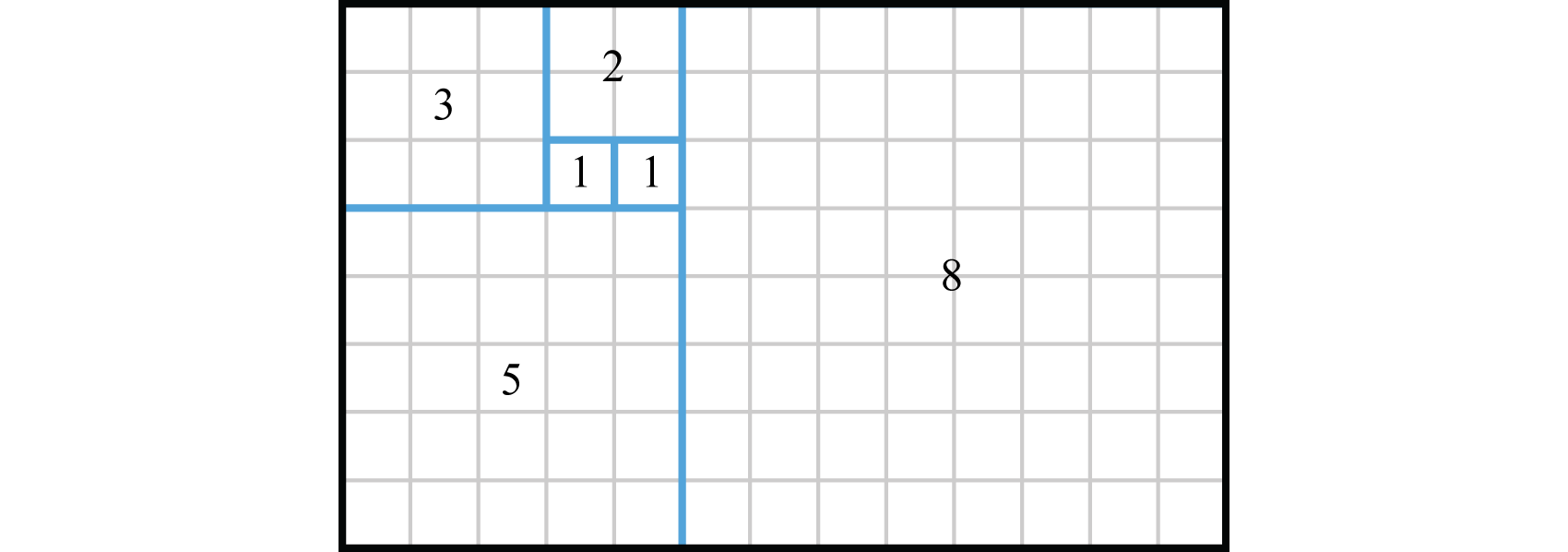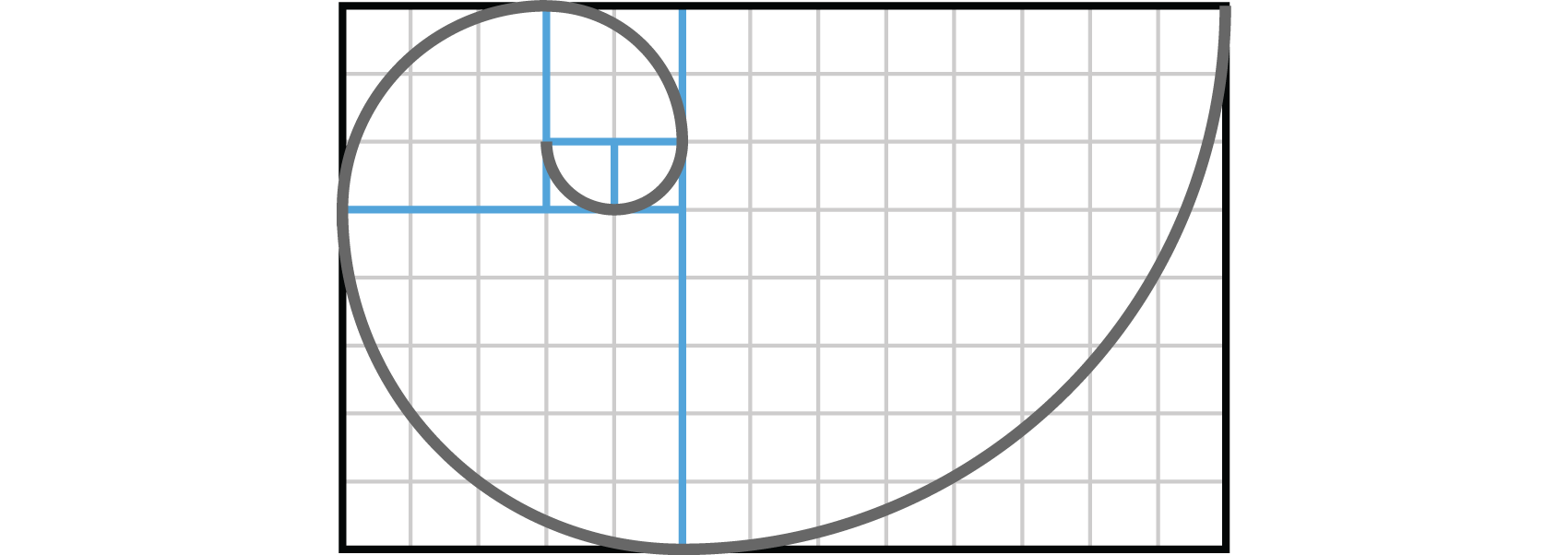This is “Introduction to Sequences and Series”, section 9.1 from the book Advanced Algebra (v. 1.0). For details on it (including licensing), click here.

Has this book helped you? Consider passing it on:
Creative Commons supports free culture from music to education. Their licenses helped make this book available to you.
DonorsChoose.org helps people like you help teachers fund their classroom projects, from art supplies to books to calculators.

## 9.1 Introduction to Sequences and Series

### Learning Objectives

1. Find any element of a sequence given a formula for its general term.
2. Use sigma notation and expand corresponding series.
3. Distinguish between a sequence and a series.
4. Calculate the nth partial sum of sequence.

## Sequences

A sequenceA function whose domain is a set of consecutive natural numbers starting with 1. is a function whose domain is a set of consecutive natural numbers beginning with 1. For example, the following equation with domain ${1,2,3,…}$ defines an infinite sequenceA sequence whose domain is the set of natural numbers ${1,2,3,…}.$:

$a(n)=5n−3 or an=5n−3$

The elements in the range of this function are called terms of the sequence. It is common to define the nth term, or the general term of a sequenceAn equation that defines the nth term of a sequence commonly denoted using subscripts $an.$, using the subscritped notation $an$, which reads “a sub n.” Terms can be found using substitution as follows:

$General term: an=5n−3First term (n=1):a1=5(1)−3=2Second term (n=2):a2=5(2)−3=7Third term (n=3):a3=5(3)−3=12Fourth term (n=4):a3=5(4)−3=17Fifth term (n=5):a3=5(5)−3=22 ⋮$

This produces an ordered list,

$2,7,12,17,22,…$

The ellipsis (…) indicates that this sequence continues forever. Unlike a set, order matters. If the domain of a sequence consists of natural numbers that end, such as ${1,2,3,…,k}$, then it is called a finite sequenceA sequence whose domain is ${1,2,3,…,k}$ where k is a natural number..

### Example 1

Given the general term of a sequence, find the first 5 terms as well as the 100th term: $an=n(n−1)2.$

Solution:

To find the first 5 terms, substitute 1, 2, 3, 4, and 5 for n and then simplify.

$a1=1(1−1)2=1(0)2=02=0a2=2(2−1)2=2(1)2=22=1a3=3(3−1)2=3(2)2=62=3a4=4(4−1)2=4(3)2=122=6a5=5(5−1)2=5(4)2=202=10$

Use $n=100$ to determine the 100th term in the sequence.

$a100=100(100−1)2=100(99)2=9,9002=4,950$

Answer: First five terms: 0, 1, 3, 6, 10; $a100=4,950$

Sometimes the general term of a sequence will alternate in sign and have a variable other than n.

### Example 2

Find the first 5 terms of the sequence: $an=(−1)nxn+1.$

Solution:

Here we take care to replace n with the first 5 natural numbers and not x.

$a1=(−1)1x1+1=−x2a2=(−1)2x2+1=x3a3=(−1)3x3+1=−x4a4=(−1)4x4+1=x5a5=(−1)5x5+1=−x6$

Answer: $−x2,x3,−x4,x5,−x6$

Try this! Find the first 5 terms of the sequence: $an=(−1)n+12n.$

Answer: 2, −4, 8, −16, 32.

One interesting example is the Fibonacci sequence. The first two numbers in the Fibonacci sequence are 1, and each successive term is the sum of the previous two. Therefore, the general term is expressed in terms of the previous two as follows:

$Fn=Fn−2+Fn−1$

Here $F1=1$, $F2=1$, and $n>2.$ A formula that describes a sequence in terms of its previous terms is called a recurrence relationA formula that uses previous terms of a sequence to describe subsequent terms..

### Example 3

Find the first 7 Fibonacci numbers.

Solution:

Given that $F1=1$ and $F2=1$, use the recurrence relation $Fn=Fn−2+Fn−1$ where $n$ is an integer starting with $n=3$ to find the next 5 terms:

$F3=F3−2+F3−1=F1+F2=1+1=2F4=F4−2+F4−1=F2+F3=1+2=3F5=F5−2+F5−1=F3+F4=2+3=5F6=F6−2+F6−1=F4+F5=3+5=8F7=F7−2+F7−1=F5+F6=5+8=13$

Answer: 1, 1, 2, 3, 5, 8, 13

Figure 9.1Leonardo Fibonacci (1170–1250) Wikipedia

Fibonacci numbers appear in applications ranging from art to computer science and biology. The beauty of this sequence can be visualized by constructing a Fibonacci spiral. Consider a tiling of squares where each side has a length that matches each Fibonacci number:Connecting the opposite corners of the squares with an arc produces a special spiral shape.This shape is called the Fibonacci spiral and approximates many spiral shapes found in nature.

## Series

A seriesThe sum of the terms of a sequence. is the sum of the terms of a sequence. The sum of the terms of an infinite sequence results in an infinite seriesThe sum of the terms of an infinite sequence denoted $S∞.$, denoted $S∞.$ The sum of the first n terms in a sequence is called a partial sumThe sum of the first n terms in a sequence denoted $Sn.$, denoted $Sn.$ For example, given the sequence of positive odd integers 1, 3, 5,… we can write:

$S∞=1+3+5+7+9+⋯ Infinite seriesS5=1+3+5+7+9=25 5th partial sum$

### Example 4

Determine the 3rd and 5th partial sums of the sequence: 3,−6, 12,−24, 48,…

Solution:

$S3=3+(−6)+12=9S5=3+(−6)+12+(−24)+48=33$

Answer: $S3=9$; $S5=33$

If the general term is known, then we can express a series using sigmaA sum denoted using the symbol $Σ$ (upper case Greek letter sigma). (or summationUsed when referring to sigma notation.) notation:

$S∞=Σn=1∞ n2=12+22+32+… Infinite seriesS3=Σn=13 n2=12+22+32 3rd partial sum$

The symbol $Σ$ (upper case Greek letter sigma) is used to indicate a series. The expressions above and below indicate the range of the index of summationThe variable used in sigma notation to indicate the lower and upper bounds of the summation., in this case represented by n. The lower number indicates the starting integer and the upper value indicates the ending integer. The nth partial sum $Sn$ can be expressed using sigma notation as follows:

$Sn=Σk=1n ak=a1+a2+⋯+an$

This is read, “the sum of $ak$ as k goes from 1 to n.” Replace n with ∞ to indicate an infinite sum.

### Example 5

Evaluate: $Σk=15(−3)n−1$.

$Σk=15(−3)k−1=(−3)1−1+(−3)2−1+(−3)3−1+(−3)4−1+(−3)5−1=(−3)0+(−3)1+(−3)2+(−3)3+(−3)4=1−3+9−27+81=61$

When working with sigma notation, the index does not always start at 1.

### Example 6

Evaluate: $Σk=25(−1)k(3k)$.

Solution:

Here the index is expressed using the variable k, which ranges from 2 to 5.

$Σk=25(−1)k(3k)=(−1)2(3⋅2)+(−1)3(3⋅3)+(−1)4(3⋅4)+(−1)5(3⋅5)=6−9+12−15=−6$

Try this! Evaluate: $Σn=15(15−9n)$.

Infinity is used as the upper bound of a sum to indicate an infinite series.

### Example 7

Write in expanded form: $Σn=0∞nn+1$.

Solution:

In this case we begin with $n=0$ and add three dots to indicate that this series continues forever.

$Σn=0∞nn+1=00+1+11+1+22+1+33+1+⋯=01+12+23+34+⋯=0+12+23+34+⋯$

Answer: $0+12+23+34+⋯$

When expanding a series, take care to replace only the variable indicated by the index.

### Example 8

Write in expanded form: $Σi=1∞(−1)i−1x2i$.

Solution:

$Σi=1∞(−1)i−1x2i=(−1)1−1x2(1)+(−1)2−1x2(2)+(−1)3−1x2(3)+⋯=(−1)0x2(1)+(−1)1x2(2)+(−1)2x2(3)+⋯=x2−x4+x6−⋯$

Answer: $x2−x4+x6−⋯$

### Key Takeaways

• A sequence is a function whose domain consists of a set of natural numbers beginning with 1. In addition, a sequence can be thought of as an ordered list.
• Formulas are often used to describe the nth term, or general term, of a sequence using the subscripted notation $an.$
• A series is the sum of the terms in a sequence. The sum of the first n terms is called the nth partial sum and is denoted $Sn.$
• Use sigma notation to denote summations in a compact manner. The nth partial sum, using sigma notation, can be written $Sn=Σk=1n ak$. The symbol $Σ$ denotes a summation where the expression below indicates that the index k starts at 1 and iterates through the natural numbers ending with the value n above.

### Part A: Sequences

Find the first 5 terms of the sequence as well as the 30th term.

1. $an=2n$

2. $an=2n+1$

3. $an=n2−12$

4. $an=n2n−1$

5. $an=(−1)n(n+1)2$

6. $an=(−1)n+1n2$

7. $an=3n−1$

8. $an=2n−2$

9. $an=(12)n$

10. $an=(−13)n$

11. $an=(−1)n−13n−1$

12. $an=2(−1)nn+5$

13. $an=1+1n$

14. $an=n2+1n$

Find the first 5 terms of the sequence.

1. $an=2x2n−1$

2. $an=(2x)n−1$

3. $an=xnn+4$

4. $an=x2nx−2$

5. $an=n x2nn+1$

6. $an=(n+1)xnn2$

7. $an=(−1)nx3n$

8. $an=(−1)n−1xn+1$

Find the first 5 terms of the sequence defined by the given recurrence relation.

1. $an=an−1+5$ where $a1=3$

2. $an=an−1−3$ where $a1=4$

3. $an=3an−1$ where $a1=−2$

4. $an=−2an−1$ where $a1=−1$

5. $an=nan−1$ where $a1=1$

6. $an=(n−1)an−1$ where $a1=1$

7. $an=2an−1−1$ where $a1=0$

8. $an=3an−1+1$ where $a1=−1$

9. $an=an−2+2an−1$ where $a1=−1$ and $a2=0$

10. $an=3an−1−an−2$ where $a1=0$ and $a2=2$

11. $an=an−1−an−2$ where $a1=1$ and $a2=3$

12. $an=an−2+an−1+2$ where $a1=−4$ and $a2=−1$

Find the indicated term.

1. $an=2−7n$; $a12$

2. $an=3n−8$; $a20$

3. $an=−4(5)n−4$; $a7$

4. $an=6(13)n−6$; $a9$

5. $an=1+1n$; $a10$

6. $an=(n+1)5n−3$; $a5$

7. $an=(−1)n22n−3$; $a4$

8. $an=n(n−1)(n−2)$; $a6$